# Test: Partnership 3

## 20 Questions MCQ Test Quantitative Techniques for CLAT | Test: Partnership 3

Description
This mock test of Test: Partnership 3 for Quant helps you for every Quant entrance exam. This contains 20 Multiple Choice Questions for Quant Test: Partnership 3 (mcq) to study with solutions a complete question bank. The solved questions answers in this Test: Partnership 3 quiz give you a good mix of easy questions and tough questions. Quant students definitely take this Test: Partnership 3 exercise for a better result in the exam. You can find other Test: Partnership 3 extra questions, long questions & short questions for Quant on EduRev as well by searching above.
QUESTION: 1

### Two friends invested Rs.1500 and Rs. 2500 in a business. They earned a profit of Rs. 800. One-half of the profit was divided equally between them and the other half was divided in proportion to their capitals. How much did each of them receive ?

Solution: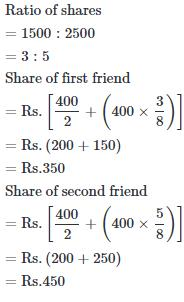QUESTION: 2

### Three persons stared a placement business with a capital of Rs. 3000. B invests Rs. 600 less than A and C invests Rs. 300 less than B. What is B's share in a profit of Rs. 886 ?

Solution: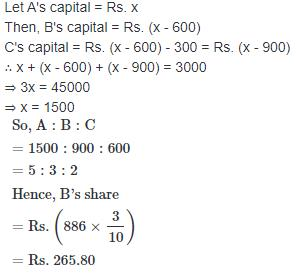QUESTION: 3

### X and Y are partners in a business. They invest in the ratio 5 : 6, at the end of 8 months X withdraws his capital. If they receive profits in the ratio of 5 : 9. Find how long Y's investment was used ?

Solution: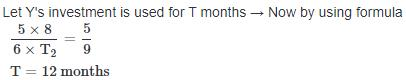QUESTION: 4

Four milkmen rented a pasture. M put to graze 16 cows for 3 months and N 20 cows for 4 months, O 18 cows for 6 months and P 42 cows for 2 months. If M's share of rent be Rs. 2400, the rent paid by O is ?

Solution:

M graze 16 cows for 3 months.
N graze 20 cows for 4 months.
O graze 18 cows for 6 months.
P graze 42 cows for 2 months.
So, Ration of Rent
= M : N : O : P
= (16 × 3) : (20 × 4) : (18 × 6) : (42 × 2)
= 48 : 80 : 108 : 84
= 12 : 20 : 27 : 21
According to the question,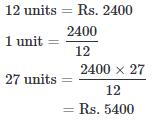QUESTION: 5

Shankar starts a business with an investment of Rs. 120000. After three months, Aniket joined him with an invesment of Rs. 190000. They earned a profit of Rs. 1750000 after one year. What is Aniket's share in the profit ?

Solution: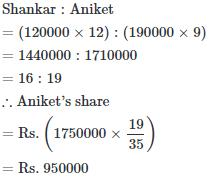QUESTION: 6

Arun started a business investing Rs. 38000. After 5 months Bakul joined him with a capital of Rs. 55000. At the end of the year the total profit was Rs. 22000. What is the approximate difference between the share of profits Arun and Bakul ?

Solution: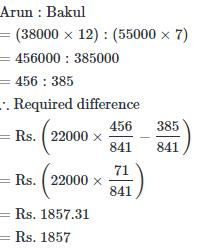QUESTION: 7

Goutam started a business with a sum of Rs. 60000. Jatin joined him 8 months later with a sum of Rs. 35000. At what respective ratio will the two share of profit after two years ?

Solution:

Shankar : Aniket
=(6000×12):(35000×8)
=720000:280000
=18:7

QUESTION: 8

Simran started a software business by investing Rs. 50000. After six months, Nanda joined her with a capital of Rs. 80000. What was Simran's share in the profit ?

Solution: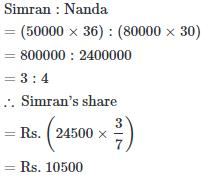QUESTION: 9

Dilip, Ram and Avtar started a shop by investing Rs. 2700, Rs. 8100 and Rs. 7200 respectively. At the end of one year, the profit earned was distributed. If Ram's share was Rs. 3600, what was their total profit ?

Solution: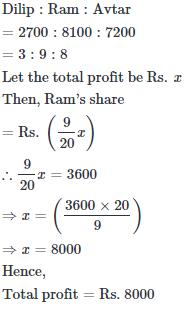QUESTION: 10

Aman started a business investing Rs. 70000. Rakhi joined him after six months with an amount of Rs. 105000 and Sagar joined them with Rs. 1.4 lakhs after another six months. The amount of profit earned should be distributed in what ratio among Aman, Rakhi and Sagar respectively, 3 years after Aman started the business ?

Solution:

Aman : Rakhi : Sagar
= (70000 × 36) : (105000 × 30) : (140000 × 24)
= 12 : 15 : 16

QUESTION: 11

A, B and C invested Rs. 47000 for more than B and B Rs. 5000 more than C, then out of total profit of Rs. 4700, C receives ?

Solution: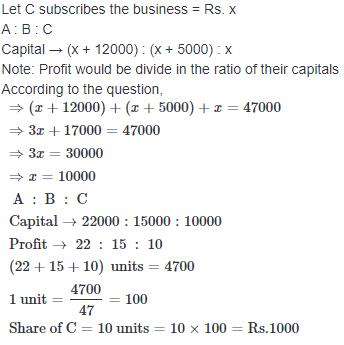QUESTION: 12

A and B started a business investing amount of Rs. 185000 and Rs. 225000 respectively. If B's share in the profit earned by them is Rs. 9000 then what is the total profit earned by them together ?

Solution: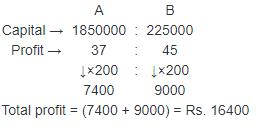QUESTION: 13

A and B stared a boutique investing amounts of Rs. 35000 and Rs. 56000 respectively. If A's share in the profit earned by them need is Rs. 45000, then what is the total profit earned ?

Solution: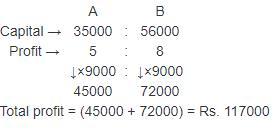QUESTION: 14

Sonia started a business investing Rs. 60000. After 6 months Vivek joined him with an amount of Rs. 140000. After 1 year Kirti also joined them with Rs. 120000. After 2 years the business yielded a total profit of Rs. 450000. What is the share of Vivek in the profit ?

Solution: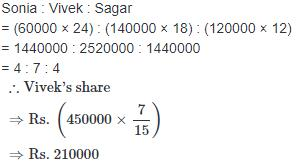QUESTION: 15

P and Q started a business in the ratio of 2 : 3. After 1 year P left the business but Q continues. After 2 years he had profit of Rs. 26000. What is the profit of Q?

Solution: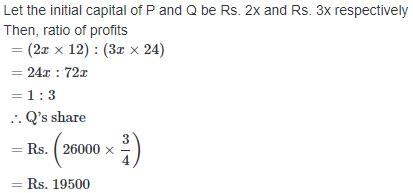QUESTION: 16

A, B and C enter into a partnership. A invests some money at the beginning, B invests double the amount after six months and C invest thrice the amount after eight months. If the annual profit be Rs. 27000; C's share (in Rs.) is ?

Solution: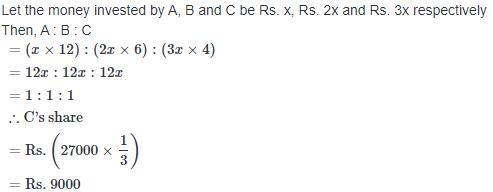QUESTION: 17

X and Y enter into a partnership with capitals in the ratio 5 : 6 and at the end of 8 months, X withdraws. If they receive profit in the ratio of 5 : 9. Find how long Y's capital was used.

Solution: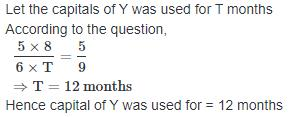QUESTION: 18

Two partners invest Rs. 125000 and Rs. 85000 respectively in a business and agree that 60% of the profit should be divided equally between them and the remaining profit is to be divided into ratio of their capitals. If one partner gets Rs. 300 more than the other. Find the total profit made in the business ?

Solution: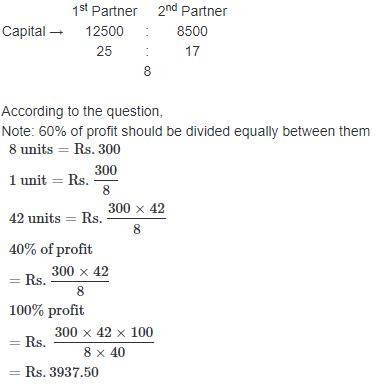QUESTION: 19

Two brother invested Rs. 50000 and Rs. 70000 respectively in a business and agreed that 70% of the profits should be divided equally between them and the remaining profit in the ratio of investment. If one brothers gets Rs. 90 more than the other. Find the total profit made in the business ?

Solution: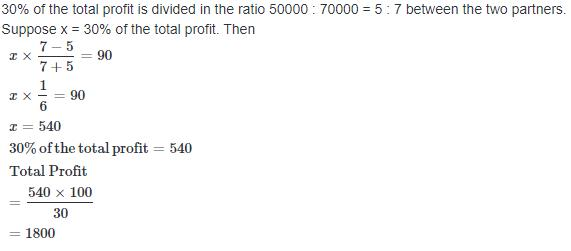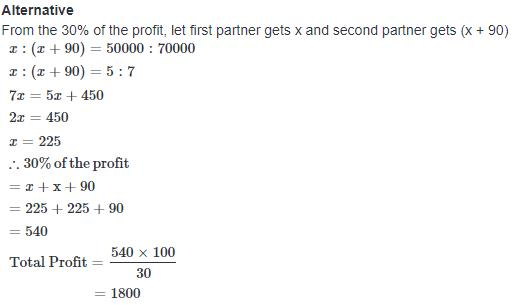QUESTION: 20

A, B and C enter into a partnership. They invest Rs. 40000, Rs. 80000 and Rs. 120000 respectively. At the end of the first year, B withdraws Rs. 40000, while at the end of the second year, C withdraws Rs. 80000. In what ratio will the profit be shared at the end of 3 years ?

Solution:

A : B : C
(40000 × 36) :(80000 × 12 + 40000 × 24) : (120000 × 24 + 40000 × 12)
= 144 : 192 : 336
= 3 : 4 : 7